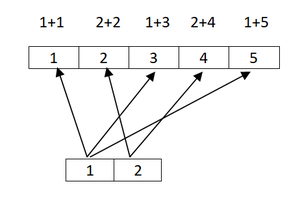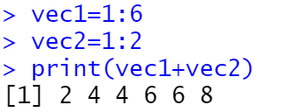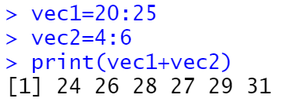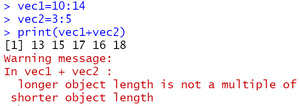Open in App
Not now

# Vector recycling in R

• Last Updated : 07 Dec, 2021

We can see vector recycling, when we perform some kind of operations like addition, subtraction. . . .etc on two vectors of unequal length. The vector with a small length will be repeated as long as the operation completes on the longer vector. If we perform an addition operation on a vector of equal length the first value of vector1 is added with the first value of vector 2 like that. The below image demonstrated operation on unequal vectors and operation on equal vector.So, the repetition of small length vector as long as completion of operation on long length vector is known as vector recycling. This is the special property of vectors is available in R language. Let us see the implementation of vector recycling.

Example 1 :

## R

 `# creating vector with``# 1 to 6 values``vec1=1:6` `# creating vector with 1:2``# values``vec2=1:2` `# adding vector1 and vector2``print``(vec1+vec2)`

Output :In vector recycling, the length of the long length vector should be the multiple of the length of a small length vector. If not we will get a warning that longer object length is not a multiple of shorter object length. Here the longer object length is multiple of the shortest object length. So, we didn’t get a warning message.

Example 2 :

## R

 `# creating vector with 20``# to 25 values``vec1=20:25` `# creating vector with 4 to``# 6 values``vec2=4:6` `# adding vector1 and vector2``print``(vec1+vec2)`

Output :Here also the longer object length is multiple of the shortest object length. So, we didn’t get warning message.

Example 3 :

## R

 `# creating vector with 10 to 14 values``vec1=10:14` `# creating vector with 3 to 5 values``vec2=3:5` `# adding vector1 and vector2``print``(vec1+vec2)`

Output :Here the longer object length is not multiple of the shortest object length. So, we got a warning message.

My Personal Notes arrow_drop_up